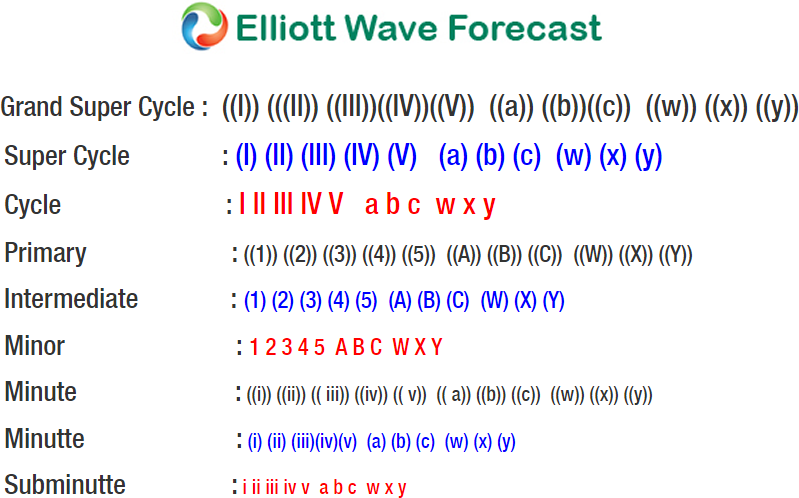# Elliott Wave Analysis: Further Strength in USDJPY

USDJPY short term Elliott Wave view suggests that the decline to 104.54 low on March 26 ended Intermediate wave (3). Wave (4) correction is in progress as a double three Elliott Wave Structure. A double three is a 7 swing corrective structure with WXY label. In the case of USDJPY, minor wave W of (4) ended at 107.01 and minor wave X of (4) ended at 105.62. As near term pullback stays above there, expect pair to extend higher within wave Y of (4).

Subdivision of Minor wave W of (4) in USDJPY unfolded as a zigzag Elliott Wave structure where Minute wave ((a)) ended at 105.9, Minute wave ((b)) ended at 105.29, and Minute wave ((c)) of W ended at 107.01. Subdivision of Minor wave X of (4) also unfolded as a zigzag Elliott Wave structure where Minute wave ((a)) ended at 106.09, Minute wave ((b)) ended at 106.45, and Minute wave ((c)) of X ended at 105.62.

Minor wave Y of (4) is currently in progress as a double three Elliott Wave structure where the rally from 105.62 ended at 107.49 in Minute wave ((w)). Expect pair to pullback within Minute wave ((x)) in 3, 7, or 11 swing to correct cycle from 4/3 low before the rally resumes.

#### USDJPY Elliott Wave 1 Hour ChartSource : https://elliottwave-forecast.com/forex/elliott-wave-analysis-strength-usdjpy/